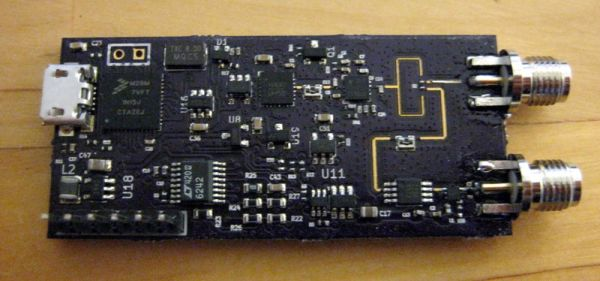# Introduction

I’ve for some time now wanted to do more RF design. Although I have taken some RF design courses, I haven’t actually made a single RF design before. But you can’t learn without doing and inspired by the MIT coffee can radar designed by Gregory Charvat, I figured that building a radar should be a doable project that would offer some challenge while also having some real world use.The simplest radar is a continuous wave Doppler radar, which continuously transmit a constant frequency signal. This signal reflects from a moving target and Doppler shift causes reflected signal to change frequency. This reflected signal is then received and mixed with the transmitted signal. Mixer product is the difference of the frequencies which is proportional to the speed of the target. This kind of radar is very simple to make, in fact there are even some children’s toys. Unfortunately it can’t detect the range of the target and isn’t that exiting.

A little more sophisticated radar which can detect also the range can be made by modulating the frequency of the transmitted signal. This kind of radar is called continuous wave frequency modulated radar (FMCW radar).

Radar transmits a chirp, which increases linearly with the frequency. This chirp is then radiated with the antenna, it reflects from the target and is received by the receiving antenna. On the reception side received and undelayed copy transmitted chirps go to the mixer. Because received chirp reflected from the target, but copy of the transmitted chirp is undelayed there is delay between the signals. This causes mixer output to have a low frequency signal which frequency depends on the distance to the target. When there are several targets the output signal is sum of different frequencies and the distances to the targets can be recovered with Fourier transform.

READ  HC-12 433MHz wireless serial communication module configuration

Glossary of the terms in the block diagram:

• MCU – Microcontroller
• VCO – Voltage controller oscillator
• PA – Power amplifier
• LNA – Low noise amplifier
• ADC – Analog to digital converter
• FFT – Fast Fourier transform

Transmitted signal is: f(t)=sin(2π(f0+f1trampt+f0)t) and the received signal is a delayed copy of it, f(t−td). Mixer multiplies these signals, outputting two frequencies which are the sum and difference of the multiplied signals. These can be solved using the trigonometric identity: sinϕsinθ=12(cos(ϕ−θ)−cos(ϕ+θ)). Sum frequency is too high to be detected and is filtered out. Frequency of the difference term gives: fdiff=td(f1−f0)tramp. Since electromagnetic waves travel at the speed of light delay of the received signal is twice the distance to the target divided by the speed of light. Distance to the target in terms of the detector frequency can be solved to be: d=cfdifftramp2(f1−f0).

For example with f0 = 6 GHz, f1 = 6.1 GHz, tramp = 5 ms, d = 100 m the output detector output frequency is 13.3 kHz, which is low enough to be detected with very cheap equipment. Even a PC soundcard would have enough bandwidth and this is actually how the MIT coffee can radar works.

How about the Doppler shift? It can be found out in two ways. If the frequency waveform is sawtooth wave, then we can take multiple consecutive range measurements and then take another FFT over them. Because movement of the target also changes the distance to it speed can be recovered and distance corrected.

Another way is to modulate the frequency with triangle wave. Doppler shift causes the mixer output frequency to be different depending on whether the frequency is increasing or decreasing.

READ  Connect a signal without distortion – via a Fujitsu relay

For more detail: 6 GHz frequency modulated radar